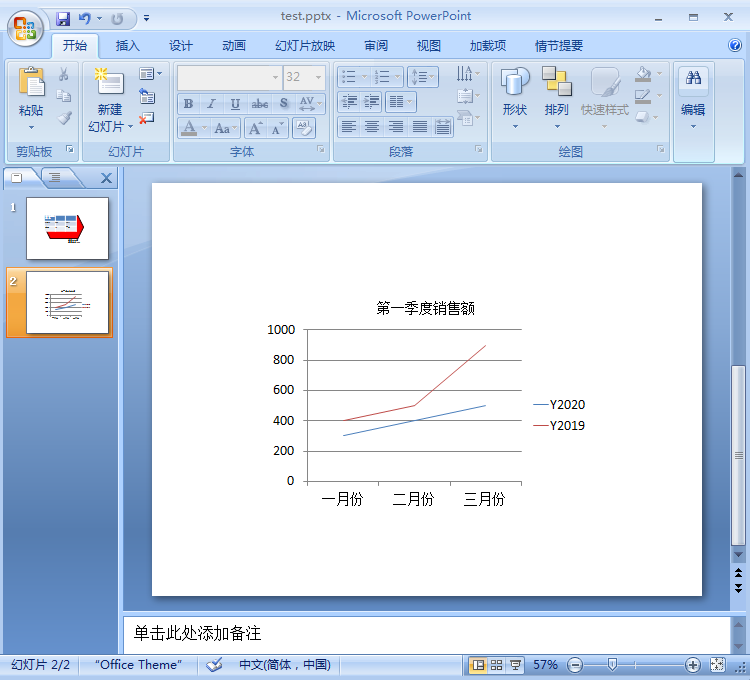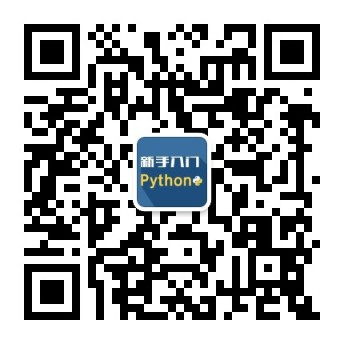2022
05-17

# 零Python基础也能驾驭的PPT自动化教程（如何使用Python实现PPT自动化）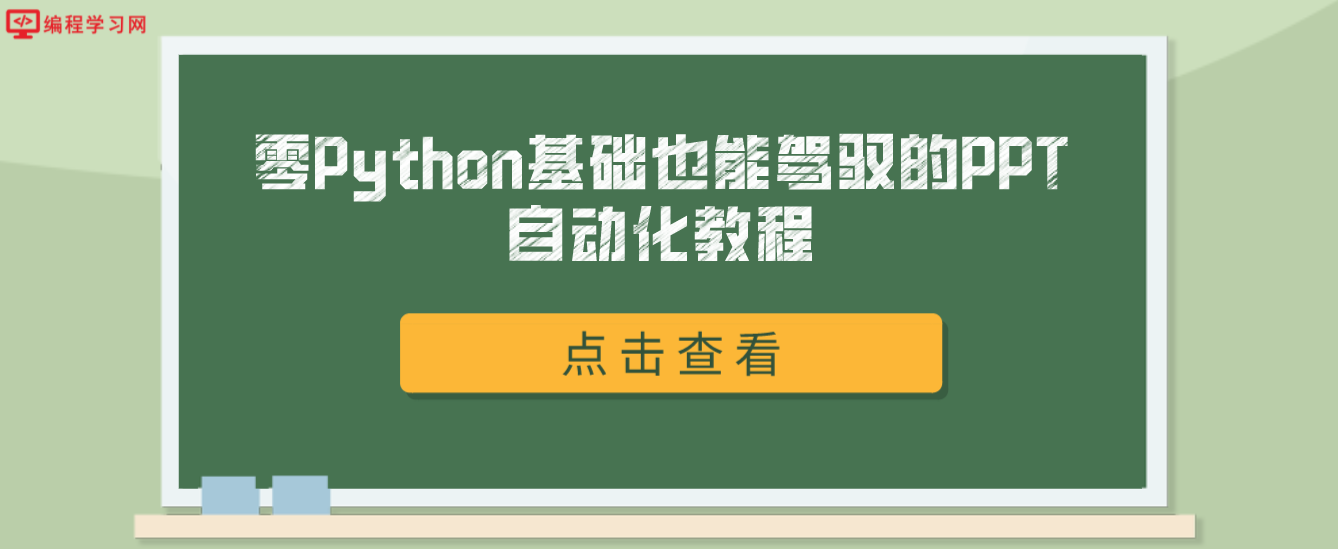#### 1.1 安装

python-pptx 是 Python 的第三方库，使用前需要通过以下命令进行安装：

`pip install python-pptx`

#### 1.2 使用步骤

`import pptx`

`prs=pptx.Presentation('test.pptx')`

`import pptx prs=pptx.Presentation('test.pptx') slide=prs.slides.add_slide(prs.slide_layouts) `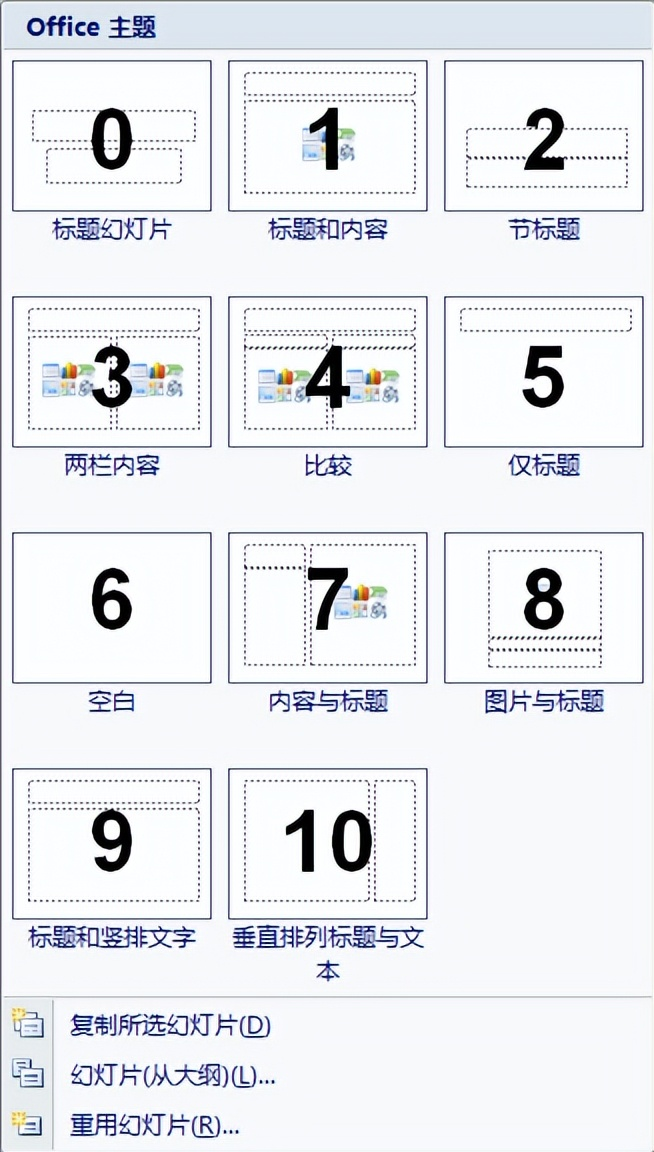```import pptx
prs = pptx.Presentation('test.pptx')
...省略部分代码
prs.save('test.pptx')```

#### 2. python-pptx 操作演示文稿写入11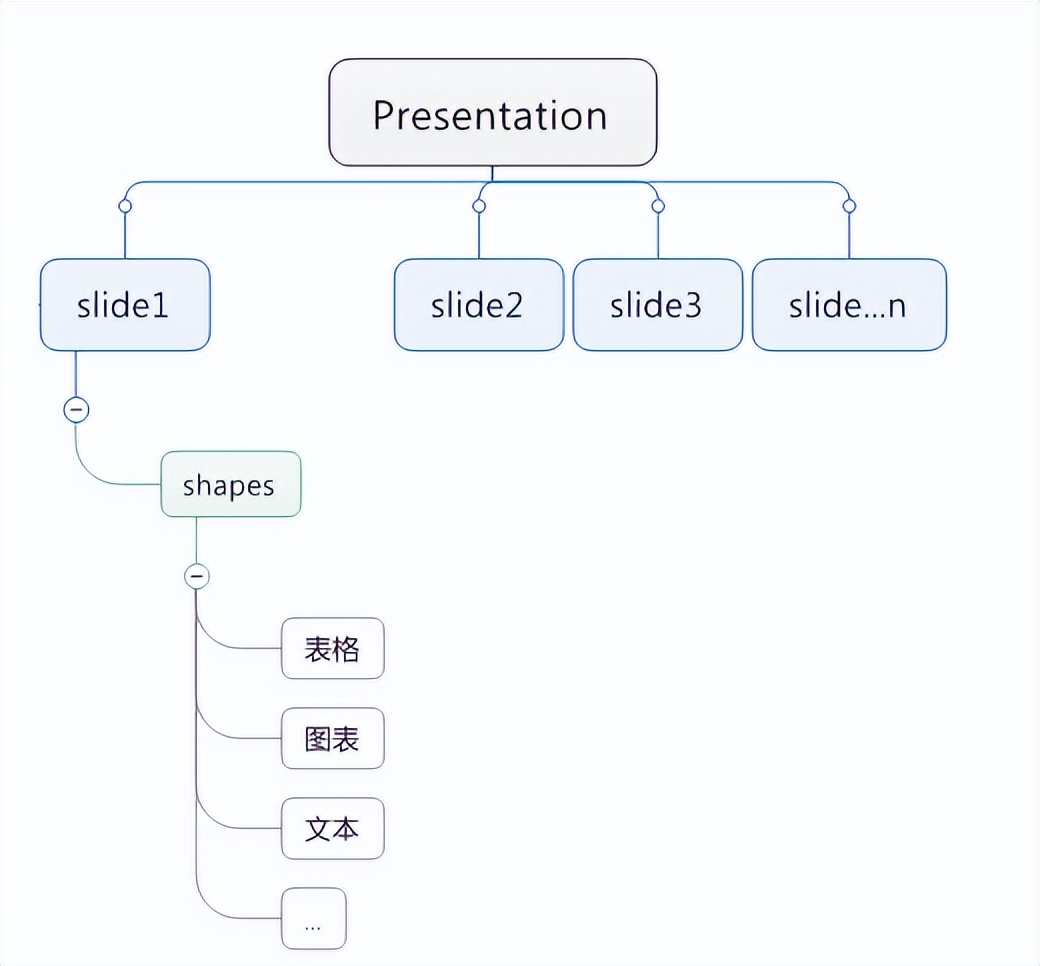#### 2.1 添加文本

```import pptx
prs=pptx.Presentation('test.pptx')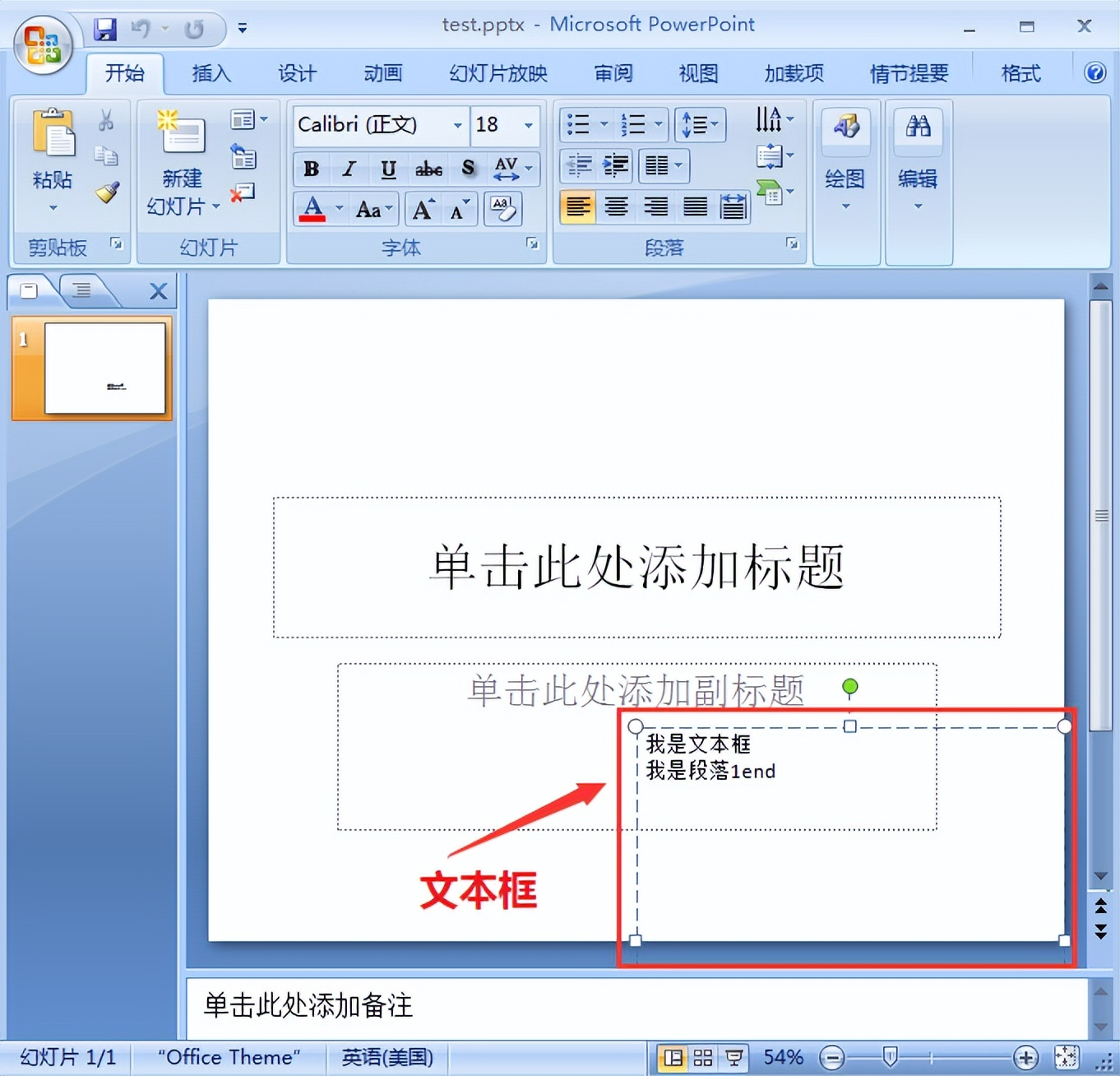#### 2.2 添加图形

```...省略部分代码 #添加自选图形 shape=slide.shapes.add_shape(MSO_SHAPE.HEXAGON,Inches(2),Inches(2),Inches(5),Inches(3)) #填充、边框 fill=shape.fill
fill.solid()
fill.fore_color.rgb=RGBColor(255,0,0)
line=shape.line
line.color.rgb=RGBColor(55,3,5)
line.width=Pt(2)
prs.save('test.pptx')```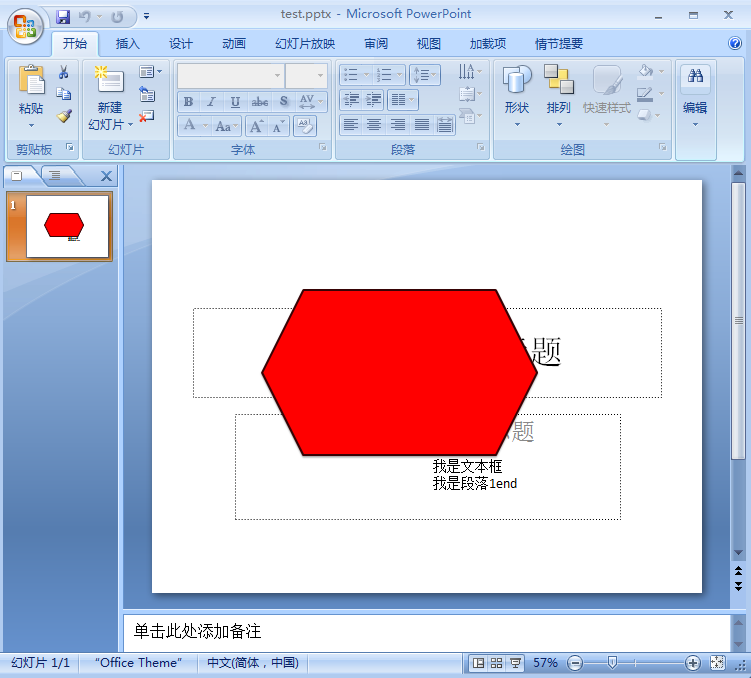#### 2.3 添加表格

```...省略部分代码
#添加表格 table=slide.shapes.add_table(3,3,Inches(2),Inches(2),Inches(4),Inches(2)).table #填充内容 table.cell(1,0).text='name' table.cell(1,1).text='age' table.cell(1,2).text='class' table.cell(2,0).text='张三' table.cell(2,1).text='19' table.cell(2,2).text='一班' #合并单元格
cell=table.cell(0,0)
cell1=table.cell(0,2)
cell.merge(cell1) table.cell(0,0).text='班级学生信息' #第一行
cell.split()#取消合并
prs.save('test.pptx')```#### 2.4 添加图表

```...省略部分代码 #写入图表 #创建一张新的幻灯片 slide1=prs.slides.add_slide(prs.slide_layouts) #定义图表数据 chart_data=CategoryChartData()